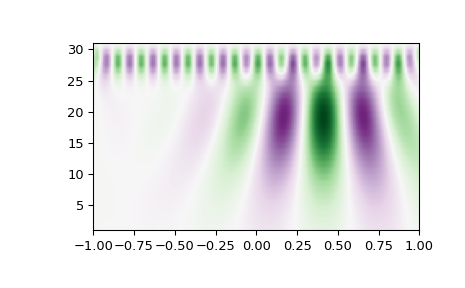# scipy.signal.cwt¶

scipy.signal.cwt(data, wavelet, widths, dtype=None, **kwargs)[source]

Continuous wavelet transform.

Performs a continuous wavelet transform on data, using the wavelet function. A CWT performs a convolution with data using the wavelet function, which is characterized by a width parameter and length parameter. The wavelet function is allowed to be complex.

Parameters
data(N,) ndarray

data on which to perform the transform.

waveletfunction

Wavelet function, which should take 2 arguments. The first argument is the number of points that the returned vector will have (len(wavelet(length,width)) == length). The second is a width parameter, defining the size of the wavelet (e.g. standard deviation of a gaussian). See ricker, which satisfies these requirements.

widths(M,) sequence

Widths to use for transform.

dtypedata-type, optional

The desired data type of output. Defaults to float64 if the output of wavelet is real and complex128 if it is complex.

New in version 1.4.0.

kwargs

Keyword arguments passed to wavelet function.

New in version 1.4.0.

Returns
cwt: (M, N) ndarray

Will have shape of (len(widths), len(data)).

Notes

New in version 1.4.0.

For non-symmetric, complex-valued wavelets, the input signal is convolved with the time-reversed complex-conjugate of the wavelet data .

length = min(10 * width[ii], len(data))
cwt[ii,:] = signal.convolve(data, np.conj(wavelet(length, width[ii],
**kwargs))[::-1], mode='same')


References

1

S. Mallat, “A Wavelet Tour of Signal Processing (3rd Edition)”, Academic Press, 2009.

Examples

>>> from scipy import signal
>>> import matplotlib.pyplot as plt
>>> t = np.linspace(-1, 1, 200, endpoint=False)
>>> sig  = np.cos(2 * np.pi * 7 * t) + signal.gausspulse(t - 0.4, fc=2)
>>> widths = np.arange(1, 31)
>>> cwtmatr = signal.cwt(sig, signal.ricker, widths)
>>> plt.imshow(cwtmatr, extent=[-1, 1, 1, 31], cmap='PRGn', aspect='auto',
...            vmax=abs(cwtmatr).max(), vmin=-abs(cwtmatr).max())
>>> plt.show()#### Previous topic

scipy.signal.morlet2

#### Next topic

scipy.signal.argrelmin